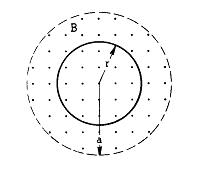# Loop in changing magnetic fieldA spatially uniform magnetic field directed out of the page is confined to a cylindrical region of space of radius a as shown above. The strength of the magnetic field increases at a constant rate such that B = Bo + Ct, where Bo and C are constants and t is time. A circular conducting loop of radius r and resistance R is placed perpendicular to the magnetic field.

a. Indicate on the diagram above the direction of the induced current in the loop. Explain your choice.
b. Derive an expression for the induced current in the loop.
c. Derive an expression for the magnitude of the induced electric field at any radius r < a.
d. Derive an expression for the magnitude of the induced electric field at any radius r > a.

I got cw for part a. The field is increasing so the loop is trying to create a field going into the page in it and so the current is cw.

I need somone(s) to take me through the rest of the problem.

What equation should I use for part b? (stuck already... :( )

Edit: I went back to the problem and this is what I did for part b:Still not sure how to do part c and part d. My equation sheet doesn't have anything for induced electric field and the comcept is still fuzzy to me. Someone(s) please explain.

Last edited:

b) remember the EMF (or voltage drop) caused by a changing flux is:
$$\xi = - \frac{d\Phi}{dt}$$
$$\xi= - \frac{d(\beta \bullet Area)}{dt}$$
$$\xi = - \frac{d[(Bo + Ct) \bullet Area)]}{dt}$$
$$\xi= - C \bullet \pi r^{2}$$

From here, you can use Ohm's law (V=IR) to solve for the current in the loop.

Don't worry too much about the negative sign, it is there to make the direction of the voltage drop is correct. Ultimatley it tells you the direction the induced current will flow, however you already found that using Lenz's law in a).

c) You need to use Faraday's law. The Electric field will be perpendicular to the magnetic field and tangent to the path of current (ie. tangent to the path of the circle). This is because according to Lenz's law, the induced electric field neds to be able to create a magnetic field opposing the original change in B.

From above:
$$\xi = - \frac{d\Phi}{dt}$$

You also know that:
$$V = \int E \bullet dl$$
But since the length we are trying to calculate the voltage drop over is a closed loop, this becomes:
$$V = \oint E \bullet dl$$

Equating these 2 equations yields Faraday's law:
$$\oint E \bullet dl = - \frac{d\Phi}{dt}$$

By symetry, we can assume that the electric field generated along the wire is constant along the circle. We also already calculated the change in flux above.
$$E \bullet (2 \pi r) = - C \bullet ( \pi r^{2})$$
$$E = - C \bullet \frac{r}{2}$$

This is true for any raduis $$\leq$$a since B is zero outside radius a.

d) if we look at a point outside of the circle where r>a:
The flux passing through a circle r>a is
$$\Phi = \pi a^{2} B$$

So now:
$$\oint E \bullet dl = - \frac{d\Phi}{dt}$$
$$E \bullet (2 \pi r) = - C \bullet ( \pi a^{2})$$
$$E = - C \bullet \frac{a^{2}}{2r}$$
for r>a

Keep in mind that this is the only time that you will encounter electric field lines that form closed paths; usually they move from one point to another, but in the special case of electrical induction they form in circles.

Last edited: